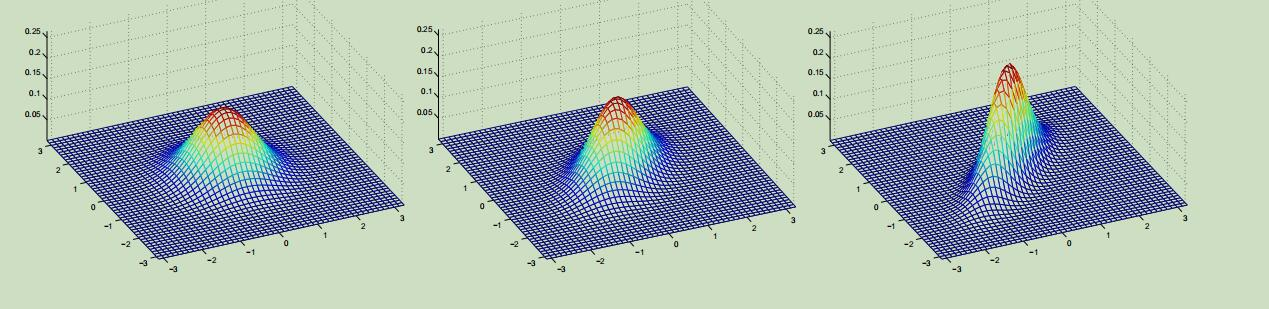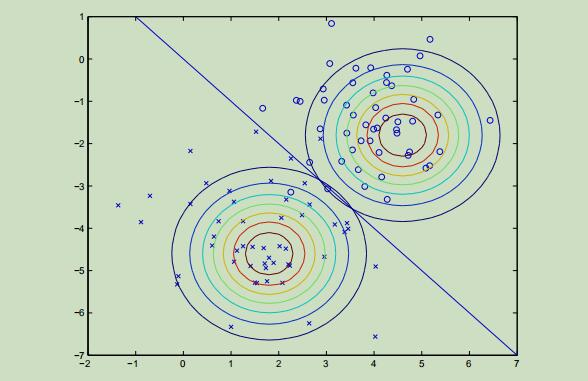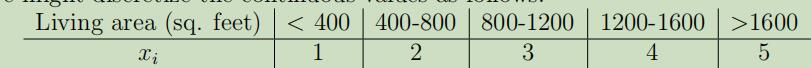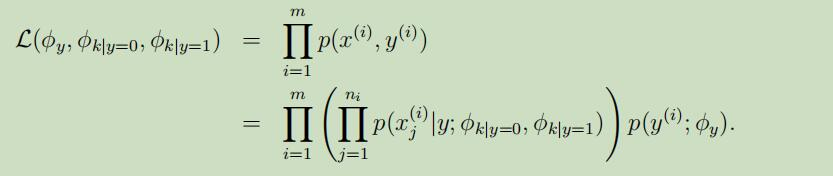# 吴恩达Stanford机器学习公开课（五）笔记

## Lecture 5 -Gaussian Discriminant Analysis. Naive Bayes.

Posted by Yunlongs on February 3, 2019

# Lecture 5 -Gaussian Discriminant Analysis. Naive Bayes.

## 多元高斯模型

1. $Cov(Z)=\mathrm{E}\left[(Z-\mathrm{E}[Z])(Z-\mathrm{E}[Z])^{T}\right]$
2. $Cov(Z)=\mathrm{E}\left[Z Z^{T}\right]-(\mathrm{E}[Z])(\mathrm{E}[Z])^{T}$同样，这些例子的期望也都还是零向量，但是它们的协方差分别为：上图最左边的为标准正态分布，可以看出来随着协方差矩阵副对角线的值越来越大，在45°的方向上（即x1=x2），图像越来越像是在这个方向上被“压缩”了一般。

## 高斯判别分析模型（Gaussian Discriminant Analysis model）

GDA算法的效果图如下：## 讨论：GDA与逻辑回归GDA是比逻辑回归更强 的假设，即若数据集满足多元正态分布，那么就一定可以导出逻辑回归；但是，逻辑回归 却不一定能导出GDA。故，基于这点，在以下情况GDA模型会比逻辑回归更好： 1.满足GDA的假设，即数据集近似于多元正态分布。 2.满足多元正态，且数据集非常大时，没有其他算法比GDA更准确。当数据集很小时，仍然比逻辑回归要好。

## 朴素贝叶斯（Naive Bayes）

$\phi_{j|y=1} = p(x_j = 1|y = 1)$ $\phi_{j|y=0} = p(x_j = 1|y = 0)$ $\phi_y = p(y = 1)$## 文本分类事件模型## 高斯朴素贝叶斯

•  y=0 6 180 12 y=0 5.92 190 11 y=0 5.58 170 12 y=0 5.92 165 10 y=1 5 100 6 y=1 5.5 150 8 y=1 5.42 130 7 y=1 5.75 150 9